### 6.2Memristive Sensing Principles

The memristive sensing scheme explained below can be classified as a novel charge-discharge (time domain) technique in which the capacitance or the inductance is calculated by measuring the memristance (memductance) and thus eliminates the need for time (frequency) measurement. In fact, the memristance (in the unit of) or memductance (in the unit of S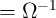) of a memristor can be simply determined by Ohm’s law, which makes the memristive sensing straightforward and fast. Furthermore, because a memristor holds the information even if its voltage/current is turned off (non-volatility), it allows for measurement circuits with low leakage power consumption and new functionalities.

#### 6.2.1Charge-Controlled Memristors

##### 6.2.1.1. Capacitance Sensing

Consider a charge-controlled memristor connected in series with a capacitor as shown in Fig. 6.1. According to Eq. 2.4 and Eq. 2.10 for the capacitance and memristance, respectively, we have(6.1)

and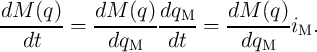(6.2)

As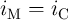, by substitutingfrom Eq. 6.2 into Eq. 6.1 we obtain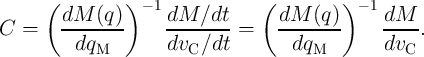(6.3)

The term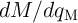is related to the intrinsic properties of the memristor. For a linear resistor this term is zero. However, it is nonzero for a memristive device or system as it is supposed to memorize the history of current flowing through the modulation in its memristance. A charge-controlled memristor with the term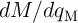being constant is suited for charge-based capacitance sensing as it can reduce the measurement to a simple memristance measurement. In fact, on one hand, as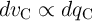and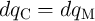, we have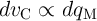. On the other hand, as for the memristor the term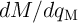is constant, we have. Therefore,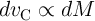and thus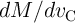is equal exactly to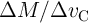. As a result, Eq. 6.3 is written as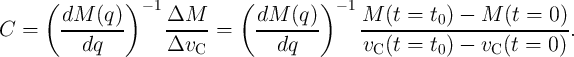(6.4)

This means that one can calculate the capacitance by measuring the modulation of the memristance and the voltage across the capacitor (or the memristor). Therefore, unlike other time domain capacitance measurement methods, memristive sensing does not need extra hardware for time/frequency measurement since the time parameter has been implicitly taken into account in the memristance modulation. Furthermore, to measure the memristance modulation, a simple circuit can be employed to switch the memristor from the MC circuit (Fig. 6.1) to a basic readout circuit employing an arbitrary resistance read method , without loosing the information during the switching due to the non-volatility of the memristor. During the readout, the memristance and the charge in the capacitor can be reset to the initial state for which the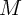and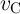are equal to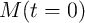and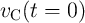, respectively. Then, for the next measurement, one should measure only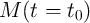and probably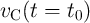if the capacitor is not fully charged (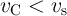).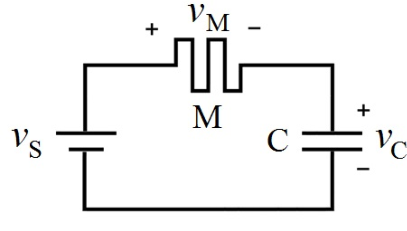Figure 6.1.: A diagram of the charge-based memristive capacitance sensing circuit.

##### 6.2.1.2. Power Monitoring

The unique ability of a memristor to record the historic profile of the voltage/current applied makes it suitable for power measurement . The total energy generated by an electric power supply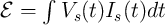, where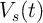is the voltage across the source and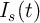is the current flowing through it. For a circuit powered by a DC voltage source (Fig. 6.2) , the energy is given by: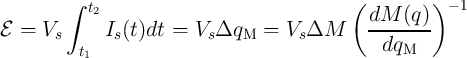(6.5)

Therefore, a charge-controlled memristor with a constant term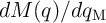reduces the power measurement to a memristance measurement. To minimize the impact of the memristor on measurements, the memristance has to be much smaller than the circuit resistance.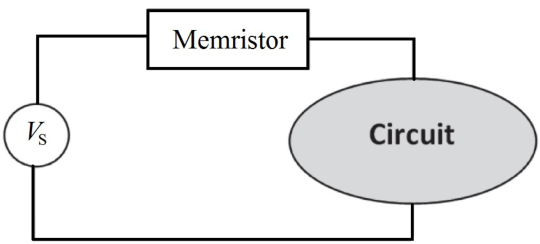Figure 6.2.: A diagram of the memristive power monitoring circuit with a charge-controlled memristor.

#### 6.2.2Flux-Controlled Memristors

##### 6.2.2.1. Inductance Sensing

According to Eq. 2.5 and Eq. 2.11 for the inductance and memductance of a flux-controlled memristor, respectively, we have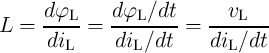(6.6)

and(6.7)

When a flux-controlled memristor is connected in parallel to an inductor (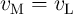in Fig. 6.3), the inductance is obtained as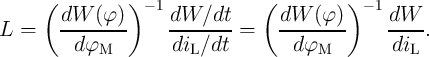(6.8)

A flux-controlled memristor with a constant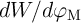term is thus suited for flux-based inductance measurement. For a common conductor the term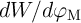is zero. However, it is nonzero for a memristive device and is related to the intrinsic properties of the memristor. Based on a similar discussion presented above for the MC circuit, in the ML circuit with a memristor with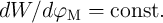, we get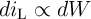. Therefore, Eq. 6.8 is simplified to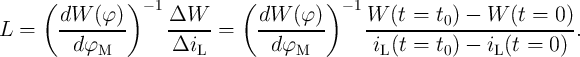(6.9)

Hence, the flux-controlled memristor in the ML circuit reduces the inductance measurement to a straightforward memductance measurement.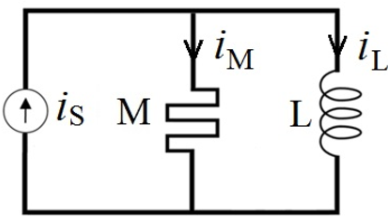Figure 6.3.: Basic memristor-inductor (ML) circuit for flux-based sensing.

##### 6.2.2.2. Power Monitoring

Similar to the charge-based power monitoring, in a circuit powered by a DC current source (Fig. 6.4) , a flux-controlled memristor with the term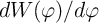being constant reduces the power measurement to a memductance measurement.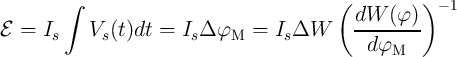(6.10)

To minimize the impact of the memristor on measurements, the memductance must be much smaller than the circuit conductance. In the following section we study different spintronic memristors which are suited for both charge- and flux-based measurements.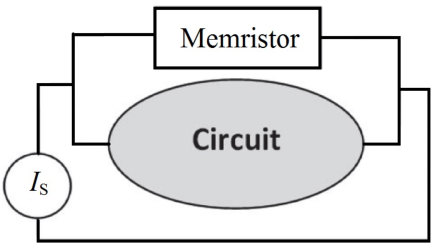Figure 6.4.: A diagram of the memristive power monitoring circuit using a flux-controlled memristor.